The Australian Journal of Mathematical Analysis and Applications

 Home News Editors Volumes RGMIA Subscriptions Authors Contact

ISSN 1449-5910

You searched for rao
Total of 59 results found in site

9: Paper Source PDF document

Paper's Title:

On the Generalized Inverse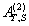over Integral Domains

Author(s):

Yaoming Yu and Guorong Wang

College of Education, Shanghai Normal University
Shanghai 200234
People's Republic of China.
yuyaoming@online.sh.cn
grwang@shnu.edu.cn

Abstract:

In this paper, we study further the generalized inverseof a matrix A over an integral domain. We give firstly some necessary and sufficient conditions for the existence of the generalized inverse, an explicit expression for the elements of the generalized inverseand an explicit expression for the generalized inverse, which reduces to the {1} inverse. Secondly, we verify that the group inverse, the Drazin inverse, the Moore-Penrose inverse and the weighted Moore-Penrose inverse are identical with the generalized inverse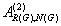for an appropriate matrix G, respectively, and then we unify the conditions for the existence and the expression for the elements of the weighted Moore-Penrose inverse, the Moore-Penrose inverse, the Drazin inverse and the group inverse over an integral domain. Thirdly, as a simple application, we give the relation between some rank equation and the existence of the generalized inverse, and a method to compute the generalized inverse. Finally, we give an example of evaluating the elements ofwithout calculating.

5: Paper Source PDF document

Paper's Title:

Some fixed point results in partial S-metric spaces

Author(s):

M. M. Rezaee, S. Sedghi, A. Mukheimer, K. Abodayeh, and Z. D. Mitrovic

Department of Mathematics, Qaemshahr Branch,
Iran.

Department of Mathematics, Qaemshahr Branch,
Iran.
E-mail: sedghi.gh@qaemiau.ac.ir

Department of Mathematics and General Sciences,
KSA.
E-mail: mukheimer@psu.edu.sa

Department of Mathematics and General Sciences,
KSA.
E-mail: kamal@psu.edu.sa

Nonlinear Analysis Research Group,
Faculty of Mathematics and Statistics,
Ton Duc Thang University, Ho Chi Minh City,
Vietnam.
E-mail: zoran.mitrovic@tdtu.edu.vn

Abstract:

We introduce in this article a new class of generalized metric spaces, called partial S-metric spaces. In addition, we also give some interesting results on fixed points in the partial S-metric spaces and some applications.

4: Paper Source PDF document

Paper's Title:

Refinement Inequalities Among Symmetric Divergence Measures

Author(s):

Inder Jeet Taneja

Departamento de Matemática,
Universidade Federal de Santa Catarina, 88.040-900
Florianópolis, Sc, Brazil

URL: http://www.mtm.ufsc.br/~taneja

Abstract:

There are three classical divergence measures in the literature on information theory and statistics, namely, Jeffryes-Kullback-Leiber’s J-divergence, Sibson-Burbea-Rao’s Jensen- Shannon divegernce and Taneja’s arithemtic - geometric mean divergence. These bear an interesting relationship among each other and are based on logarithmic expressions. The divergence measures like Hellinger discrimination, symmetric χ2divergence, and triangular discrimination are not based on logarithmic expressions. These six divergence measures are symmetric with respect to probability distributions. In this paper some interesting inequalities among these symmetric divergence measures are studied. Refinements of these inequalities are also given. Some inequalities due to Dragomir et al.  are also improved.

4: Paper Source PDF document

Paper's Title:

Scope of the Logarithmic Mean

Author(s):

Murali Rao and Agnish Dey

Department of Mathematics,
University of Florida,
Florida 32611,
U. S. A.

E-mail: mrao@ufl.edu

URL: http://people.clas.ufl.edu/mrao

E-mail: agnish@ufl.edu

URL: http://people.clas.ufl.edu/agnish

Abstract:

A number a is between two numbers x and y if and only if a is a convex combination of x and y, in other words, it is a "weighted mean" of x and y. Geometric mean, arithmetic mean are well known examples of these "means". Of more recent vintage is the logarithmic mean which has been considered in many articles in the literature. In this note, we first discuss some of its properties. Then we shall introduce the L function and explore the inverse of this function and its connection with the Lambert's Omega function.

4: Paper Source PDF document

Paper's Title:

Generalised Models for Torsional Spine Reconnection

Author(s):

Ali Khalaf Hussain Al-Hachami

Department of Mathematics,
College of Education For Pure Sciences,
Wasit University,
Iraq.
E-mail: alhachamia@uowasit.edu.iq

Abstract:

Three-dimensional (3D) null points are available in wealth in the solar corona, and the equivalent is probably going to be valid in other astrophysical situations. On-going outcomes from sun oriented perceptions and from reproductions propose that reconnection at such 3D nulls may assume a significant job in the coronal dynamics. The properties of the torsional spine method of magnetic reconnection at 3D nulls are researched. Kinematic model are created, which incorporate the term ηJ that is spatially localised around the null, stretching out along the spine of the null. The null point is to research the impact of shifting the level of asymmetry of the null point magnetic field on the subsequent reconnection process where past examinations constantly considered a non-nonexclusive radially symmetric null. Specifically we analyse the rate of reconnection of magnetic flux at the spine of null point. Logical arrangements are determined for the enduring kinematic equation, and contrasted and the after effects of torsional spine reconnection models when the current is restricted in which the Maxwell conditions are illuminated. The geometry of the current layers inside which torsional spine reconnection happen is autonomous on the symmetry of the magnetic field. Torsional spine reconnection happens in a thin cylinder around the spine, with circular cross-segment when the fan eigenvalues are extraordinary. The short axis of the circle being along the solid field bearing. Just as it was discovered that the fundamental structure of the method of attractive reconnection considered is unaffected by changing the magnetic field symmetry, that is, the plasma flow is discovered rotational around the spine of null point. The spatiotemporal pinnacle current, and the pinnacle reconnection rate achieved, are found not to rely upon the level of asymmetry.

4: Paper Source PDF document

Paper's Title:

Existence of Solution of Differential and Riemann-Liouville Equation Via Fixed Point Approach in Complex Valued b-Metric Spaces

Author(s):

K. Afassinou, A. A. Mebawondu, H. A. Abass and O. K. Narain

Department of Science Access,
South Africa.
E-mail: komia@aims.ac.za

DST-NRF Centre of Excellence in Mathematical and Statistical Sciences (CoE-MaSS),
Johannesburg,
South Africa.
E-mail: dele@aims.ac.za

DST-NRF Centre of Excellence in Mathematical and Statistical Sciences (CoE-MaSS),
Johannesburg,
South Africa.
E-mail: hammedabass548@gmail.com

School of Mathematics, Statistics and Computer Science,
University of KwaZulu-Natal, Durban,
South Africa.
E-mail: naraino@ukzn.ac.za

Abstract:

In this paper, we establish some fixed point and common fixed point results for a new type of generalized contractive mapping using the notion of C-class function in the framework of complex valued b-metric spaces. As an application, we establish the existence and uniqueness of a solution for Riemann-Liouville integral and ordinary differential equation in the framework of a complete complex valued b-metric spaces. The obtained results generalize and improve some fixed point results in the literature.

2: Paper Source PDF document

Paper's Title:

Contact With Adhesion between a Deformable Body and a Foundation

Author(s):

B. Teniou and M. Sofonea

Laboratoire de Mathematiques Appliquées et Modélisation,
Université Mentouri, Constantine 25000,
Algeria
tenioubou2@yahoo.fr

Laboratoire de Mathématiques et Physiques pour les Systémes,
Univesité de Perpignan,
France.
sofonea@univ-perp.fr

Abstract:

The aim of this work is study a dynamic contact problem between a deformable body and a foundation where the deformations are supposed to be small. The contact is with adhesion and normal compliance. The behavior of this body is modeled by a nonlinear elastic-visco-plastic law. The evolution of bonding field is described by a nonlinear differential equation. We derive a variational formulation of the contact problem and we prove the existence and uniqueness of its solution. The proof is based on the construction of three intermediate problems and then we construct a contraction mapping whose unique fixed point will be the weak solution of the mechanical problem.

2: Paper Source PDF document

Paper's Title:

Maximal Inequalities for Multidimensionally Indexed Demimartingales and the Hájek-Rényi Inequality for Associated Random Variables

Author(s):

Tasos C. Christofides and Milto Hadjikyriakou

Department of Mathematics and Statistics
University of Cyprus
P.O.Box 20537, Nicosia 1678, Cyprus

tasos@ucy.ac.cy
miltwh@gmail.com

Abstract:

Demimartingales and demisubmartingales introduced by Newman and
Wright (1982) generalize the notion of martingales and
submartingales respectively. In this paper we define
multidimensionally indexed demimartingales and demisubmartingales
and prove a maximal inequality for this general class of random
variables. As a corollary we obtain a Hájek-Rényi inequality
for multidimensionally indexed associated random variables, the bound of which, when reduced to the case of single index, is sharper than the bounds already known in the literature.

2: Paper Source PDF document

Paper's Title:

On Eigenvalues and Boundary Curvature of the C*-algebra Numerical Rang

Author(s):

M. T. Heydari

Department of Mathematics,
College of Sciences,
Yasouj University,
Yasouj, 75914-74831,
Iran.

E-mail: heydari@yu.ac.ir

Abstract:

Let A be a C*-algebra with unit 1 and a∈A be a nilpotent. By Donoghue's Theorem, all corner points of its numerical range V(a) belong to the spectrum σ(a). It is therefore natural to expect that, more generally, the distance from a point p on the boundary ∂ V(a) of V(a) to σ(a) should be in some sense bounded by the radius of curvature of ∂ V(a) at p.

2: Paper Source PDF document

Paper's Title:

Some New Inequalities of Hermite-Hadamard and Fejér Type for Certain Functions with Higher Convexity

Author(s):

Steven G. From

Department of Mathematics,
U.S.A.
E-mail: sfrom@unomaha.edu

Abstract:

In this paper, we present some new inequalities of Hermite-Hadamard or Fejér type for certain functions satisfying some higher convexity conditions on one or more derivatives.
An open problem is given also.
Some applications to the logarithmic mean are given.

2: Paper Source PDF document

Paper's Title:

Inequalities for Discrete F-Divergence Measures: A Survey of Recent Results

Author(s):

Sever S. Dragomir1,2

1Mathematics, School of Engineering & Science
Victoria University, PO Box 14428
Melbourne City, MC 8001,
Australia
E-mail: sever.dragomir@vu.edu.au

2DST-NRF Centre of Excellence in the Mathematical and Statistical Sciences,
School of Computer Science & Applied Mathematics,
University of the Witwatersrand,
Private Bag 3, Johannesburg 2050,
South Africa
URL: http://rgmia.org/dragomir

Abstract:

In this paper we survey some recent results obtained by the author in providing various bounds for the celebrated f-divergence measure for various classes of functions f. Several techniques including inequalities of Jensen and Slater types for convex functions are employed. Bounds in terms of Kullback-Leibler Distance, Hellinger Discrimination and Varation distance are provided. Approximations of the f-divergence measure by the use of the celebrated Ostrowski and Trapezoid inequalities are obtained. More accurate approximation formulae that make use of Taylor's expansion with integral remainder are also surveyed. A comprehensive list of recent papers by several authors related this important concept in information theory is also included as an appendix to the main text.

2: Paper Source PDF document

Paper's Title:

Polyanalytic Functions on Subsets of Z[i]

Author(s):

Abtin Daghighi

SE-581 83,
Sweden.

E-mail: abtindaghighi@gmail.com

Abstract:

For positive integers q we consider the kernel of the powers Lq where L is one of three kinds of discrete analogues of the Cauchy-Riemann operator. The first two kinds are well-studied, but the third kind less so. We give motivations for further study of the third kind especially since its symmetry makes it more appealing for the cases q 2.

From an algebraic perspective it makes sense that the chosen multiplication on the kernels is compatible with the choice of pseudo-powers. We propose such multiplications together with associated pseudo-powers. We develop a proof-tool in terms of certain sets of uniqueness.

2: Paper Source PDF document

Paper's Title:

A New Adomian Approach to Solving Integral Equations of Fredholm and Volterra Second Kind

Author(s):

Ouedraogo Seny, Nebie Abdoul Wassiha, Youssouf Pare, Blaise Some

Departement de mathematiques,
Universite Joseph Ki-Zerbo,
Burkina Faso.
E-mail: oseny@yahoo.fr, nebwass@yahoo.fr
pareyoussouf@yahoo.fr, some@univ-ouaga.bf

Abstract:

In order to simplify the resolution of Fredholm and Volterra's second type integral equations, we propose a new approach based on the Adomian Decompositional Method (ADM). We test the new approach on several examples with success.

2: Paper Source PDF document

Paper's Title:

Reduced Generalized Combination Synchronization Between Two n-Dimensional Integer-Order Hyperchaotic Systems and One m-Dimensional Fractional-Order Chaotic System

Author(s):

Smail Kaouache, Mohammed Salah Abdelouahab and Rabah Bououden

Laboratory of Mathematics and their interactions,
Abdelhafid Boussouf University Center, Mila.
Algeria
E-mail: smailkaouache@gmail.com, medsalah3@yahoo.fr, rabouden@yahoo.fr

Abstract:

This paper is devoted to investigate the problem of reduced generalized combination synchronization (RGCS) between two n-dimensional integer-order hyperchaotic drive systems and one m-dimensional fractional-order chaotic response system. According to the stability theorem of fractional-order linear system, an active mode controller is proposed to accomplish this end. Moreover, the proposed synchronization scheme is applied to synchronize three different chaotic systems, which are the Danca hyperchaotic system, the modified hyperchaotic Rossler system, and the fractional-order Rabinovich-Fabrikant chaotic system. Finally, numerical results are presented to fit our theoretical analysis.

1: Paper Source PDF document

Paper's Title:

New Reverses of Schwarz, Triangle and Bessel Inequalities in Inner Product Spaces

Author(s):

S. S. Dragomir

School of Computer Science and Mathematics, Victoria University of Technology, PO BOX
14428, MCMC 8001, VICTORIA, AUSTRALIA.

sever.dragomir@vu.edu.au
URL
: http://rgmia.vu.edu.au/SSDragomirWeb.html

Abstract:

New reverses of the Schwarz, triangle and Bessel inequalities in inner product spaces are pointed out. These results complement the recent ones obtained by the author in the earlier paper . Further, they are employed to establish new Grüss type inequalities. Finally, some natural integral inequalities are stated as well.

1: Paper Source PDF document

Paper's Title:

Fixed Point Theorems for a Finite Family of Asymptotically Nonexpansive Mappings

Author(s):

E. Prempeh

Department of Mathematics,
Kwame Nkrumah University of Science and Technology,
Kumasi, Ghana
edward_prempeh2000@yahoo.com

Abstract:

Let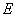be a real reflexive Banach space with a uniformly Gâteaux differentiable norm,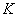be a nonempty bounded closed convex subset of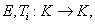i=1,2,...,r be a finite family of asymptotically nonexpansive mappings such that for each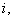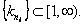Let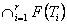be a nonempty set of common fixed points of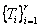and define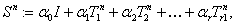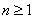. Let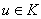be fixed and let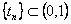be such that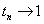as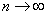. We can prove that the sequence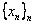satisfying the relation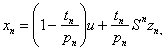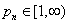associated with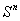, converges strongly to a fixed point of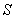providedpossesses uniform normal structure. Furthermore we prove that the iterative process: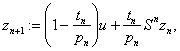, converges strongly to a fixed point of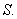1: Paper Source PDF document

Paper's Title:

Orthogonality and ε-Orthogonality in Banach Spaces

Author(s):

H. Mazaheri and S. M. Vaezpour

Faculty of Mathematics, Yazd University, Yazd, Iran
vaezpour@yazduni.ac.ir
hmazaheri@yazduni.ac.ir

Abstract:

A concept of orthogonality on normed linear space was introduced by Brickhoff, also the concept of ε-orthogonality was introduced by Vaezpour. In this note, we will consider the relation between these concepts and the dual of X. Also some results on best coapproximation will be obtained.

1: Paper Source PDF document

Paper's Title:

The Invariant Subspace Problem for Linear Relations on Hilbert Spaces

Author(s):

Daniel Grixti-Cheng

Department of Mathematics and Statistics,
The University of Melbourne,
Melbourne, VIC, 3010
Australia.
D.Grixti@ms.unimelb.edu.au

Abstract:

We consider the invariant subspace problem for linear relations on Hilbert spaces with the aim of promoting interest in the problem as viewed from the theory of linear relations. We present an equivalence between the single valued and multivalued invariant subspace problems and give some new theorems pertaining to the invariant subspace problem for linear relations on a Hilbert space.

1: Paper Source PDF document

Paper's Title:

Two Remarks on Commutators of Hardy Operator

Author(s):

Yasuo Komori-Furuya

School of High Technology for Human Welfare
Tokai University
317 Nishino Numazu, Shizuoka 410-0395 Japan
komori@wing.ncc.u-tokai.ac.jp

Abstract:

Fu and Lu showed that
the commutator of multiplication operator by b and
the n-dimensional Hardy operator
is bounded on Lp if b is in some CMO space.
We shall prove the converse of this theorem
and also prove that their result is optimal by giving a counterexample

1: Paper Source PDF document

Paper's Title:

The Square Number by the Approximation

Author(s):

Masaki Hisasue

Asahikawa Fuji Girls' High School
Asahikawa Hanasaki-cho 6-3899
Hokkaido, Japan

masaki@fuji.ed.jp

Abstract:

In this paper, we give square numbers by using the solutions of Pell's equation.

1: Paper Source PDF document

Paper's Title:

A-Normal Operators In Semi Hilbertian Spaces

Author(s):

Department of Mathematics,
College of Education for Girls in Sarat Ebeidah 61914, Abha,
King Khalid University
Saudi Arabia

Abstract:

In this paper we study some properties and inequalities of A-normal operators in semi-Hilbertian spaces by employing some known results for vectors in inner product spaces. We generalize also most of the inequalities of (α,β)-normal operators discussed in Hilbert spaces .

1: Paper Source PDF document

Paper's Title:

Szegö Limits and Haar Wavelet Basis

Author(s):

M. N. N. Namboodiri and S. Remadevi

Dept. of Mathematics, Cochin University of Science and Technology,
Cochin-21, Kerala,
India.

nambu@cusat.ac.in

Dept. of Mathematics, College of Engineering,
Cherthala, Kerala,
India.

rema@mec.ac.in

Abstract:

This paper deals with Szegö type limits for multiplication operators on L2 (R) with respect to Haar orthonormal basis. Similar studies have been carried out by Morrison for multiplication operators Tf using Walsh System and Legendre polynomials . Unlike the Walsh and Fourier basis functions, the Haar basis functions are local in nature. It is observed that Szegö type limit exist for a class of multiplication operators Tf , f∈ L (R) with respect to Haar (wavelet) system with appropriate ordering. More general classes of orderings of Haar system are identified for which the Szegö type limit exist for certain classes of multiplication operators. Some illustrative examples are also provided.

1: Paper Source PDF document

Paper's Title:

Existence and Regularity of Minima of an Integral Functional in Unbounded Domain

Author(s):

L. Aharouch, J. Bennouna and A. Bouajaja

King Khalid University
Faculty of Arts and Science Mha'l Asir
Saudi Arabia.

E-mail: laharouch@gmail.com

Université Sidi Mohammed Ben Abdellah
Faculté des Sciences Dhar-Mahraz
B.P 1796 Atlas Fčs,
Maroc.

Email: jbennouna@hotmail.com

Abstract:

We prove the existence and the regularity of minima for a functional defined on a suitable Sobolev space.

1: Paper Source PDF document

Paper's Title:

Some Grüss Type Inequalities in Inner Product Spaces

Author(s):

Sever S. Dragomir1,2

1Mathematics, School of Engineering & Science
Victoria University, PO Box 14428
Melbourne City, MC 8001,
Australia
E-mail: sever.dragomir@vu.edu.au

2School of Computer Science & Applied Mathematics,
University of the Witwatersrand,
Private Bag 3, Johannesburg 2050,
South Africa

URL: http://rgmia.org/dragomir

Abstract:

Some inequalities in inner product spaces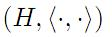that provide upper bounds for the quantities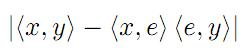and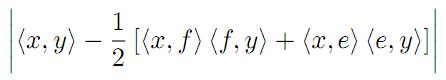,

where e,f ∈ H with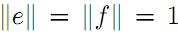and x,y are vectors in H satisfying some appropriate assumptions are given. Applications for discrete and integral inequalities are provided as well.

1: Paper Source PDF document

Paper's Title:

Extreme Curvature of Polynomials and Level Sets

Author(s):

Stephanie P. Edwards, arah J. Jensen, Edward Niedermeyer, and Lindsay Willett

Department of Mathematics,
Hope College,
Holland, MI 49423,
U.S.A.
E-mail: sedwards@hope.edu
E-mail: tarahjaye@gmail.com
E-mail: eddie.niedermeyer@gmail.com
E-mail: willettlm1@gmail.com
WWW: http://math.hope.edu/sedwards/

Abstract:

Let f be a real polynomial of degree n. Determining the maximum number of zeros of kappa, the curvature of f, is an easy problem: since the zeros of kappa are the zeros of f'', the curvature of f is 0 at most n-2 times. A much more intriguing problem is to determine the maximum number of relative extreme values for the function kappa. Since kappa'=0 at each extreme point of kappa, we are interested in the maximum number of zeros of kappa'. In 2004, the first author and R. Gordon showed that if all the zeros of f'' are real, then f has at most n-1 points of extreme curvature. We use level curves and auxiliary functions to study the zeros of the derivatives of these functions. We provide a partial solution to this problem, showing that f has at most n-1 points of extreme curvature, given certain geometrical conditions. The conjecture that f has at most n-1 points of extreme curvature remains open.

1: Paper Source PDF document

Paper's Title:

Generalized k-distance-balanced Graphs

Author(s):

Amir Hosseini and Mehdi Alaeiyan

Department of mathematics, Karaj Branch,
Iran.
E-mail: amir.hosseini@kiau.ac.ir, hosseini.sam.52@gmail.com

Department of Mathematics,
Iran University of Science and Technology, Tehran,
Iran.
E-mail: alaeiyan@iust.ac.ir

Abstract:

A nonempty graph Γ is called generalized k-distance-balanced, whenever every edge ab has the following property: the number of vertices closer to a than to b, k, times of vertices closer to b than to a, or conversely, k N .In this paper we determine some families of graphs that have this property, as well as to prove some other result regarding these graphs.

1: Paper Source PDF document

Paper's Title:

Analysis of a Frictional Contact Problem for Viscoelastic Piezoelectric Materials

Author(s):

Meziane Said Ameur, Tedjani Hadj Ammar and Laid Maiza

Departement of Mathematics,
El Oued University,
P.O. Box 789, 39000 El Oued,
Algeria.
E-mail: said-ameur-meziane@univ-eloued.dz

Departement of Mathematics,
El Oued University,
P.O. Box 789, 39000 El Oued,
Algeria.

Department of Mathematics,
Kasdi Merbah University,
30000 Ouargla,
Algeria.
E-mail: maiza.laid@univ-ouargla.dz

Abstract:

In this paper, we consider a mathematical model that describes the quasi-static process of contact between two thermo-electro-viscoelastic bodies with damage and adhesion. The damage of the materials caused by elastic deformations. The contact is frictional and modeled with a normal compliance condition involving adhesion effect of contact surfaces. Evolution of the bonding field is described by a first order differential equation. We derive variational formulation for the model and prove an existence and uniqueness result of the weak solution. The proof is based on arguments of evolutionary variational inequalities, parabolic inequalities, differential equations, and fixed point theorem.

Search and serve lasted 0 second(s).